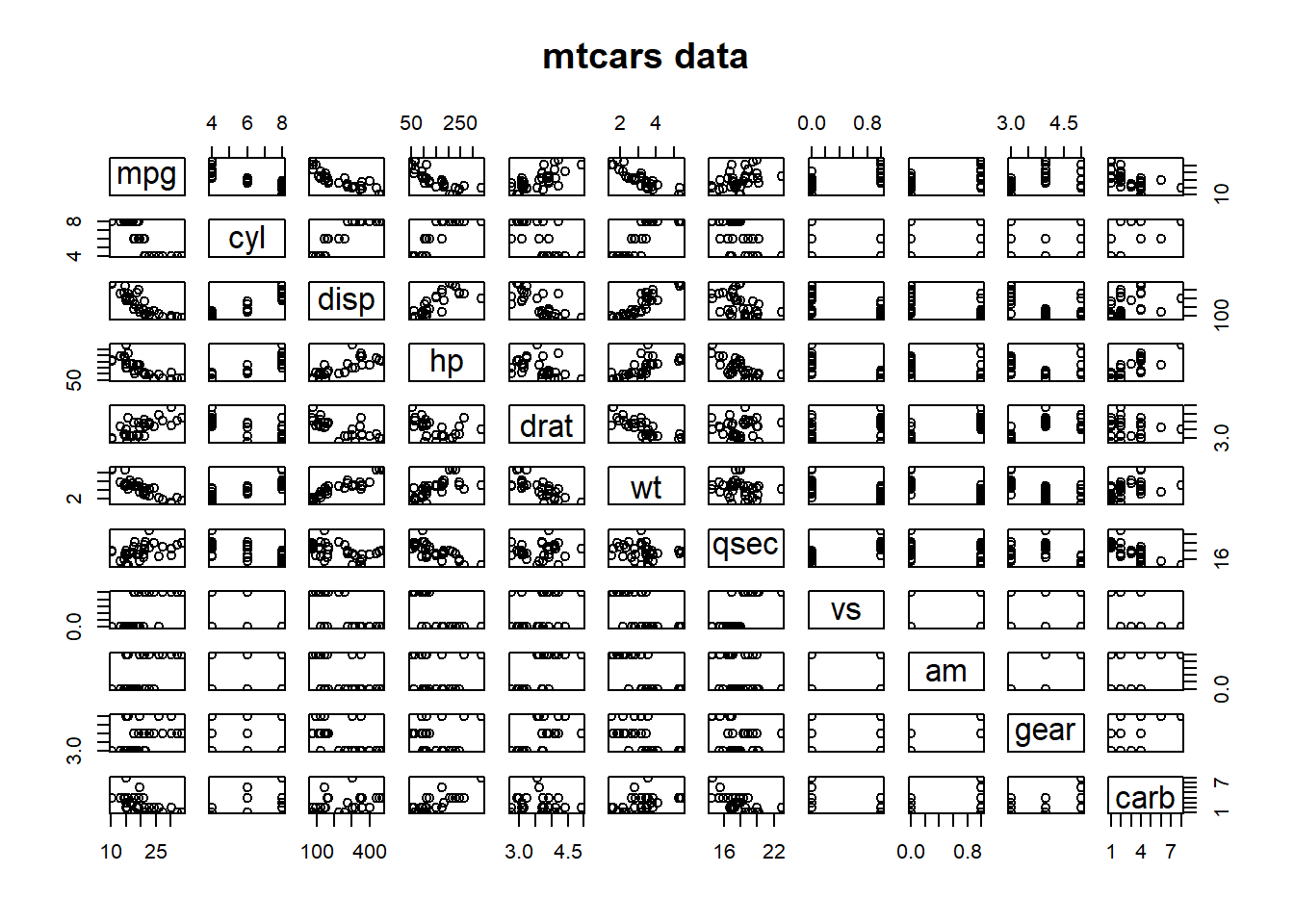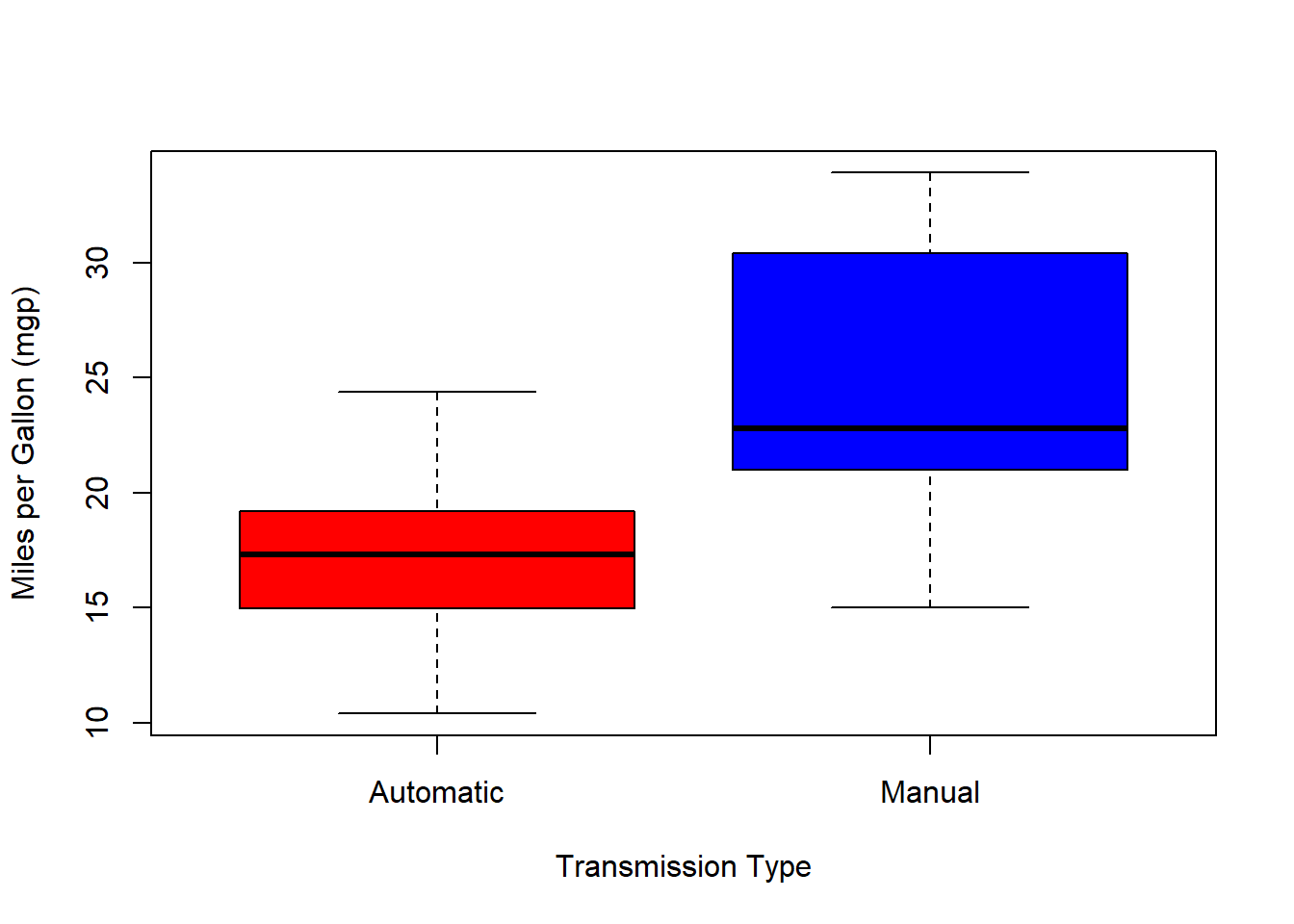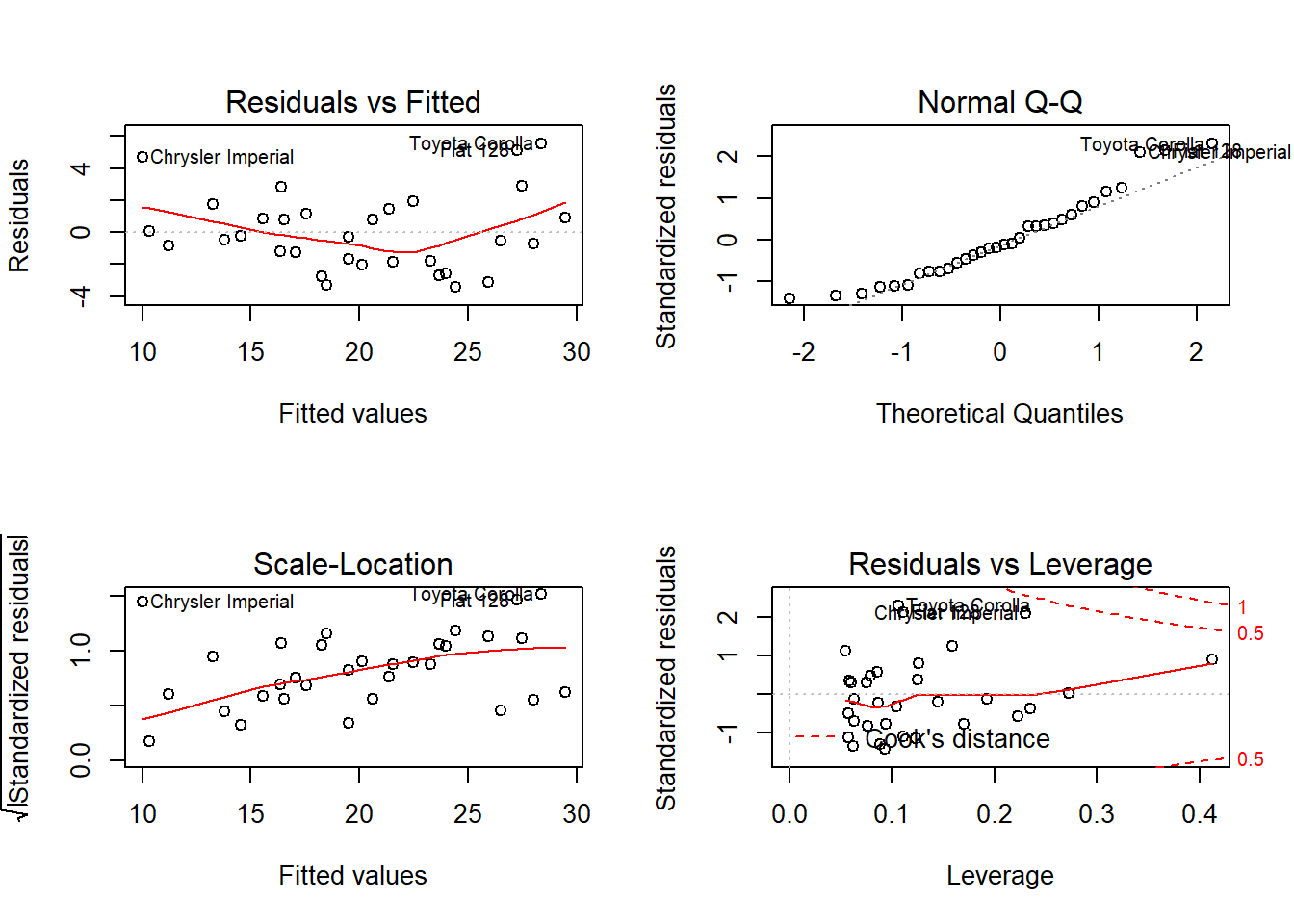## Executive Summary

This report will dive into the 1974 Motor Trend vehicle design data to determine the relationship between the type of transmission and MPG for the 1973-1974 model years.

We will first examine the data to form some hypotheses, the use several linear modeling techniques to explore the relationships in the data. We’ll be trying to answer the following:

1. Is an automatic or manual transmission better for MPG?
2. Quantify the MPG difference between automatic and manual transmissions.

## Exploratory Data Analysis

This data set contains 32 observations of 11 variables. The first thing we’ll do is plot each of the variables against each other. See figure 1.

You can see in the paired plot that there could be a relationship between the type of transmission (am) and the mpg. However, there also seems to be many other linear relationships between mpg and other variables. The number of cylinders, engine displacement, the number of carburetors, the number of forward gears and especially the weight all seem to vary relative to mpg.

First we’ll look into just the relationship between mpg and transmission type.

t.test(mpg ~ am, data = mtcars)
##
##  Welch Two Sample t-test
##
## data:  mpg by am
## t = -3.7671, df = 18.332, p-value = 0.001374
## alternative hypothesis: true difference in means is not equal to 0
## 95 percent confidence interval:
##  -11.280194  -3.209684
## sample estimates:
## mean in group 0 mean in group 1
##        17.14737        24.39231

As you can see in Figure 2 there is a clear separation in the means of the Automatic Transmission cars and the Manual Transmission cars. Running the t-test on these two variables alone yields a p-value of 0.001374 seeming to show there is a very strong relationship between the transmission type and fuel economy.

## Regression Analysis

We know from the t-test that there is a significant difference in mpg between the automatic and manual transmission types. Now we’ll fit a linear model to determine how much of the variance is explained by the model.

fit <- lm(mpg~am, data=mtcars)
summary(fit)$r.squared ##  0.3597989 Our summary of the fit of the regression shows an R^2 value of 0.3598. A large percentage of the variance is explained by the residuals. Now let’s try a multivariate regression. We’ll add independent variables two at a time then use an anova to compare them. We’ll leave out the top speed (vs) and the quarter mile time (qsec) since they are largely a function of the other variables and add the others in one at a time ## Analysis of Variance Table ## ## Model 1: mpg ~ am ## Model 2: mpg ~ am + wt ## Model 3: mpg ~ am + wt + hp ## Model 4: mpg ~ am + wt + hp + gear ## Model 5: mpg ~ am + wt + hp + gear + disp ## Model 6: mpg ~ am + wt + hp + gear + disp + carb ## Model 7: mpg ~ am + wt + hp + gear + disp + carb + drat ## Res.Df RSS Df Sum of Sq Pr(>Chi) ## 1 30 720.90 ## 2 29 278.32 1 442.58 7.662e-16 *** ## 3 28 180.29 1 98.03 0.0001488 *** ## 4 27 179.34 1 0.95 0.7087456 ## 5 26 177.93 1 1.41 0.6490651 ## 6 25 167.51 1 10.41 0.2163282 ## 7 24 163.53 1 3.99 0.4442112 ## --- ## Signif. codes: 0 '***' 0.001 '**' 0.01 '*' 0.05 '.' 0.1 ' ' 1 As you can see from our Chi-square anova test only models 2 and 3 added statistical significance to the residual sum of squares. As a result we’ll use the regression I’ve called fit 2 above that includes the transmission type, weight and horsepower. But wait! summary(fit2)$coefficients
##                Estimate  Std. Error   t value     Pr(>|t|)
## (Intercept) 34.00287512 2.642659337 12.866916 2.824030e-13
## am           2.08371013 1.376420152  1.513862 1.412682e-01
## wt          -2.87857541 0.904970538 -3.180850 3.574031e-03
## hp          -0.03747873 0.009605422 -3.901830 5.464023e-04

The model shows an increase of 2 mpg for an automatic transmission. However, when we check the p-values of the new model we can see that the transmission type’s p-value is now all the way up to .14! Taken in light of the other variables it no longer has a statistically significant effect.

With this as our final model we’ll do some residual testing. Figure 3 shows the residual testing of our model. It shows there are 3 cars which may be acting as outliers and that they have significant leverage. They are the Chrysler Imperial, Toyota Corolla and the Fiat 128. Let’s pull them out and do one more summary.

summary(lm(formula = mpg ~ am + wt + hp, data = mtcars[-c(17,18,20),]))\$r.squared
##  0.8873634

Taking them out brings our r-squared up to 89% and the transmission type becomes even more insignificant. As a result we can say that while it at first appears the transmission type is a predictor of the mpg it is in fact not and the best predictors of mpg in the dataset are the weight and horsepower of the cars.

## Appendix

### Figure 1

pairs(mtcars, main = "mtcars data")### Figure 2

boxplot(mpg~am, data = mtcars, col = c(2,4), ylab = "Miles per Gallon (mgp)", xlab = "Transmission Type", names = c("Automatic", "Manual"))### Figure 3

par(mfrow = c(2, 2))
plot(fit2)